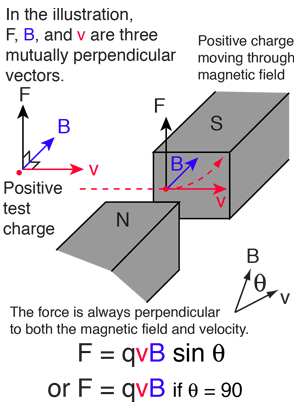# Magnetic Forces on Moving ChargesThe magnetic force on a free moving charge is perpendicular to both the velocity of the charge and the magnetic field with direction given by the right hand rule. The force is given by the charge times the vector product of velocity and magnetic field.

 Magnetic interactions with charge
 Magnetic force applications
 Bending a charge into a circular path

If the velocity is perpendicular to the magnetic field then the force is given by the simple product:

## Force = charge x velocity x B-field

For charge q = e = x 10^ C
with velocity v= x 10^ m/s
moving perpendicular to a magnetic field B = Tesla = Gauss
the force is F = x 10^ N.
If the angle between the velocity and magnetic field is degrees
the force is F = x 10^ N.

Data may be entered in any of the fields. Whey you have finished entering data, click on the quantity you wish to calculate in the active formula above. The quantities will not be forced to be consistent until you click on a choice. Default values will be entered for unspecified parameters, but all values may be changed.

Index

Electromagnetic force

Magnetic field concepts

 HyperPhysics***** Electricity and Magnetism R Nave
Go Back